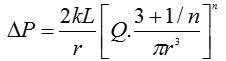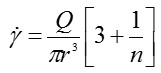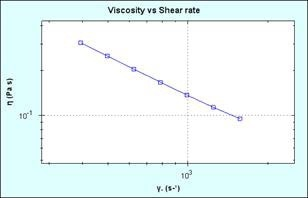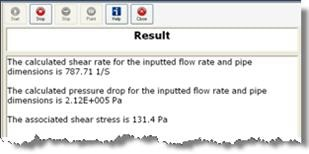# Processing Non-Newtonian Products - Determining the Pressure Drop for a Power Law Fluid Along a Straight Circular Pipe

Often in the process and chemical industries fluids have to be pumped over long distances from storage to different processing units and/or between different plant sites. Thus, it is essential to determine the pressure requirements for pumping, the selection of optimum pipe diameter and the measurement and control of flow rate. Many of the formulae needed to determine these processing parameters are available in the literature and require some knowledge of such parameters and fluid properties.

When using non-Newtonian fluids, it is often enough to consider them as power law fluids in terms of processing because of the shear rates involved.

If the liquid follows power law behavior, then the pressure drop across the pipe can be expressed by the following equation (1):(1)

where k is the consistency and n the power law index; Q is the flow rate through the pipe radius r with a pressure drop ΔP. For Newtonian fluids, the power law index is 1.

The shear rate encountered during this process is given by the following equation (2):(2)

Therefore, if the volumetric flow rate for a given pipe diameter is measured, then the shear rate encountered during the pumping process can be estimated. The value of n can be taken as 1 if it is unknown at this stage; for a Newtonian fluid, n is equal to 1. Measuring the viscosity at selected shear rates slightly below and above the measured value enables a relevant portion of the flow curve to be generated. Then, a power law model can be fitted to the data, and values of n and k can be calculated. The values of n and k can be used in equations 1 and 2 to obtain the ΔP across the pipe and the true shear rate, respectively. These relationships assume steady state (fully developed) laminar flow and no slip conditions at the pipe walls.

## Experimental

• This experiment concerns a shampoo product being transported via a straight circular pipe with a length of 10 m and radius of 0.0125 m. The power law index was known to be 0.15, and the volumetric flow rate is 0.0005 m3/second.
• Rotational rheometer measurements were carried out using a Kinexus rotational rheometer with a Peltier plate cartridge and a cone-plate measuring system, and using standard pre-configured sequences in the rSpace software.
• A standard loading sequence was employed to subject both samples to a consistent and controllable loading protocol.
• All rheology measurements were taken at 25 °C.
• The relevant shear rate for flow in the pipe was automatically determined as part of the test sequence by employing input values of length, pipe radius, power law index and volumetric flow rate.
• A shear rate table using a start value of (calculated shear rate/2) and an end value of (calculated shear rate ×2) was made, and a power law model fitted to the resultant flow curve and the calculated pressure drop was determined.

## Results and Discussion

Based on the information provided, the calculated shear rate for flow in the pipe was determined to be 787 s-1. This automatically generated a table of shear rates between 394 s-1 and 1578 s-1, and produced a shear thinning curve, which is shown in Figure 1.Figure 1. Viscosity vs. shear rate plot (on log axes) for a shampoo over the calculated shear rate range

A power law analysis on the resultant curve produced values of n and k of 0.1506 and 48.7, respectively. Then, these values were employed to calculate the true shear rate (if n was unknown initially), the associated shear stress, and the pressure drop. Later, these calculated values were displayed as a prompt in the rSpace software, as shown in Figure 2.Figure 2. Calculated values for pressure drop, shear rate, and shear stress displayed as a prompt

A pressure difference across the pipe of 212 kPa and an associated shear stress of 131.4 Pa are needed to pump this material at the required flow rate.

## Conclusion

A shear rate value was determined from the input values of pipe dimensions and flow rate, which were employed to plot a flow curve. Then, equation 1 was used to calculate the pressure drop across the pipe by using the parameters obtained from a power law analysis of the curve. Therefore, this sequence is useful to predict pressure requirements in order to achieve the required flow rate in a straight circular pipe.

References

A Handbook of Elementary Rheology; HA Barnes

Non-Newtonian Flow in the Process Industries; RP Chaabra & JF Richardson

• Note that testing is recommended to be undertaken with cone and plate or parallel plate geometry – with the latter being preferred for dispersions and emulsions with large particle sizes. Such material types may also require the use of serrated or roughened geometries to avoid artefacts relating to slippage at the geometry surface.This information has been sourced, reviewed and adapted from materials provided by Malvern Panalytical.

For more information on this source, please visit Malvern Panalytical.

## Citations

Please use one of the following formats to cite this article in your essay, paper or report:

• APA

Malvern Panalytical. (2019, September 03). Processing Non-Newtonian Products - Determining the Pressure Drop for a Power Law Fluid Along a Straight Circular Pipe. AZoM. Retrieved on September 15, 2019 from https://www.azom.com/article.aspx?ArticleID=13728.

• MLA

Malvern Panalytical. "Processing Non-Newtonian Products - Determining the Pressure Drop for a Power Law Fluid Along a Straight Circular Pipe". AZoM. 15 September 2019. <https://www.azom.com/article.aspx?ArticleID=13728>.

• Chicago

Malvern Panalytical. "Processing Non-Newtonian Products - Determining the Pressure Drop for a Power Law Fluid Along a Straight Circular Pipe". AZoM. https://www.azom.com/article.aspx?ArticleID=13728. (accessed September 15, 2019).

• Harvard

Malvern Panalytical. 2019. Processing Non-Newtonian Products - Determining the Pressure Drop for a Power Law Fluid Along a Straight Circular Pipe. AZoM, viewed 15 September 2019, https://www.azom.com/article.aspx?ArticleID=13728.

## Ask A Question

Do you have a question you'd like to ask regarding this article?